2020/08/01 12:11

#Relation Net 是 CVPR2018的一篇论文，论文链接：

https://arxiv.org/pdf/1711.06025.pdf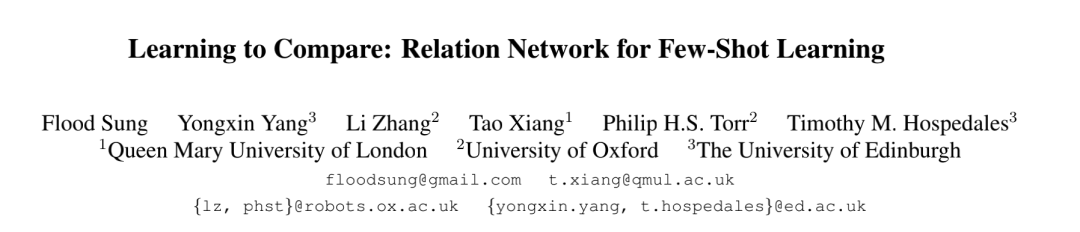Relation Network由 embedding model 和 relation model 组成。Relation Network 的核心思想是：首先通过embedding model分别提取 support set 和 testing set中图像的特征图，然后将特征图中代表通道数的维度进行拼接，得到一个新的特征图。然后把新的特征图送入 relation model 进行运算得到 relation score，这个值代表了两张图的相似度。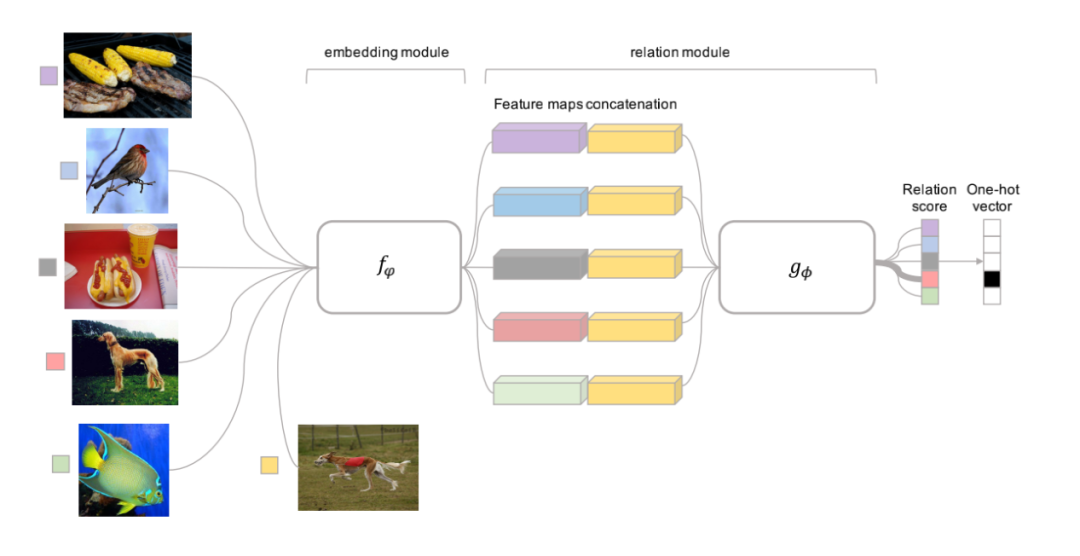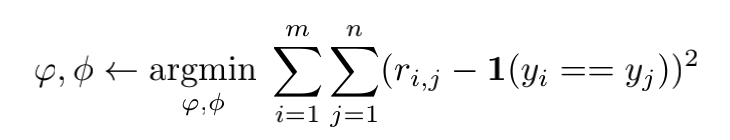下载安装命令

## CPU版本安装命令

## GPU版本安装命令
pip install -f https://paddlepaddle.org.cn/pip/oschina/gpu paddlepaddle-gpu

Relation Network

1. 搭建 Relation Network 网络

class BaseNet:
def conv_bn_layer(self,
input,
num_filters,
filter_size,
stride=1,
groups=1,
act=None,
name=None,
data_format='NCHW'):
n = filter_size * filter_size * num_filters
conv = fluid.layers.conv2d(
input=input,
num_filters=num_filters,
filter_size=filter_size,
stride=stride,
groups=groups,
act=None,
param_attr=ParamAttr(name=name + "_weights", initializer=fluid.initializer.Normal(0,math.sqrt(2. / n))),
bias_attr=ParamAttr(name=name + "_bias",
initializer=fluid.initializer.Constant(0.0)),
name=name + '.conv2d.output.1',
data_format=data_format)

bn_name = "bn_" + name

return fluid.layers.batch_norm(
input=conv,
act=act,
momentum=1,
name=bn_name + '.output.1',
param_attr=ParamAttr(name=bn_name + '_scale',
initializer=fluid.initializer.Constant(1)),
bias_attr=ParamAttr(bn_name + '_offset',
initializer=fluid.initializer.Constant(0)),
moving_mean_name=bn_name + '_mean',
moving_variance_name=bn_name + '_variance',
data_layout=data_format)



• Input：传入待卷积处理的张量对象；

• num_filter：卷积核数量(输出的卷积结果的通道数)；

• filter_size：卷积核尺寸；

• stride：卷积步长；

• groups：分组卷积的组数量；

• act:接在 BN 层后的激活函数，如果为 None，则不使用激活函数；

• name:在运算图中的对象名称。

class EmbeddingNet(BaseNet):
def net(self,input):
conv = self.conv_bn_layer(
input=input,
num_filters=64,
filter_size=3,
act='relu',
name='embed_conv1')
conv = fluid.layers.pool2d(
input=conv,
pool_size=2,
pool_stride=2,
pool_type='max')
conv = self.conv_bn_layer(
input=conv,
num_filters=64,
filter_size=3,
act='relu',
name='embed_conv2')
conv = fluid.layers.pool2d(
input=conv,
pool_size=2,
pool_stride=2,
pool_type='max')
conv = self.conv_bn_layer(
input=conv,
num_filters=64,
filter_size=3,
act='relu',
name='embed_conv3')
conv = self.conv_bn_layer(
input=conv,
num_filters=64,
filter_size=3,
act='relu',
name='embed_conv4')
return conv



Relation model 代码部分如下：

class RelationNet(BaseNet):
def net(self, input, hidden_size):
conv = self.conv_bn_layer(
input=input,
num_filters=64,
filter_size=3,
act='relu',
name='rn_conv1')
conv = fluid.layers.pool2d(
input=conv,
pool_size=2,
pool_stride=2,
pool_type='max')
conv = self.conv_bn_layer(
input=conv,
num_filters=64,
filter_size=3,
act='relu',
name='rn_conv2')
conv = fluid.layers.pool2d(
input=conv,
pool_size=2,
pool_stride=2,
pool_type='max')
fc = fluid.layers.fc(conv,size=hidden_size,act='relu',
param_attr=ParamAttr(name='fc1_weights',
initializer=fluid.initializer.Normal(0,0.01)),
bias_attr=ParamAttr(name='fc1_bias',
initializer=fluid.initializer.Constant(1)),
)
fc = fluid.layers.fc(fc, size=1,act='sigmoid',
param_attr=ParamAttr(name='fc2_weights',
initializer=fluid.initializer.Normal(0,0.01)),
bias_attr=ParamAttr(name='fc2_bias',
initializer=fluid.initializer.Constant(1)),
)
return fc



sample_image = fluid.layers.data('sample_image', shape=[3, 84, 84], dtype='float32')
query_image = fluid.layers.data('query_image', shape=[3, 84, 84], dtype='float32')

sample_query_image = fluid.layers.concat([sample_image, query_image], axis=0)
sample_query_feature = embed_model.net(sample_query_image)



sample_batch_size = fluid.layers.shape(sample_image)
query_batch_size = fluid.layers.shape(query_image)



sample_feature = fluid.layers.slice(
sample_query_feature,
axes=,
starts=,
ends=[sample_batch_size])
if k_shot > 1:
# few_shot
sample_feature = fluid.layers.reshape(sample_feature, shape=[c_way, k_shot, 64, 19, 19])
sample_feature = fluid.layers.reduce_sum(sample_feature, dim=1)
query_feature = fluid.layers.slice(
sample_query_feature,
axes=,
starts=[sample_batch_size],
ends=[sample_batch_size + query_batch_size])



sample_feature_ext = fluid.layers.unsqueeze(sample_feature, axes=0)
query_shape = fluid.layers.concat(
[query_batch_size, fluid.layers.assign(np.array([1, 1, 1,1]).astype('int32'))])
sample_feature_ext = fluid.layers.expand(sample_feature_ext, query_shape)



query_feature_ext = fluid.layers.unsqueeze(query_feature, axes=0)
if k_shot > 1:
sample_batch_size = sample_batch_size / float(k_shot)
sample_shape = fluid.layers.concat(
[sample_batch_size, fluid.layers.assign(np.array([1, 1, 1, 1]).astype('int32'))])
query_feature_ext = fluid.layers.expand(query_feature_ext, sample_shape)



query_feature_ext = fluid.layers.transpose(query_feature_ext, [1, 0, 2, 3, 4])
relation_pairs = fluid.layers.concat([sample_feature_ext, query_feature_ext], axis=2)
relation_pairs = fluid.layers.reshape(relation_pairs, shape=[-1, 128, 19, 19])



relation = RN_model.net(relation_pairs, hidden_size=8)
relation = fluid.layers.reshape(relation, shape=[-1, c_way])



one_hot_label = fluid.layers.one_hot(query_label, depth=c_way)
loss = fluid.layers.square_error_cost(relation, one_hot_label)
loss = fluid.layers.reduce_mean(loss)



2. 训练策略

episode based training的实现步骤如下：

1. 训练需要循环迭代 N 个 episode,每1个 episode 会在 training set 中随机选取 C 个类别的中的 K 个数据，组成1个sample set数据集。C和 K 对应 support set 中的 C-way K-shot，一共有 C x K个样本。

2. 在 C 个类别中剩余的样本中随机选取几个样本作为 query set, 进行训练。

3. 验证模型复现效果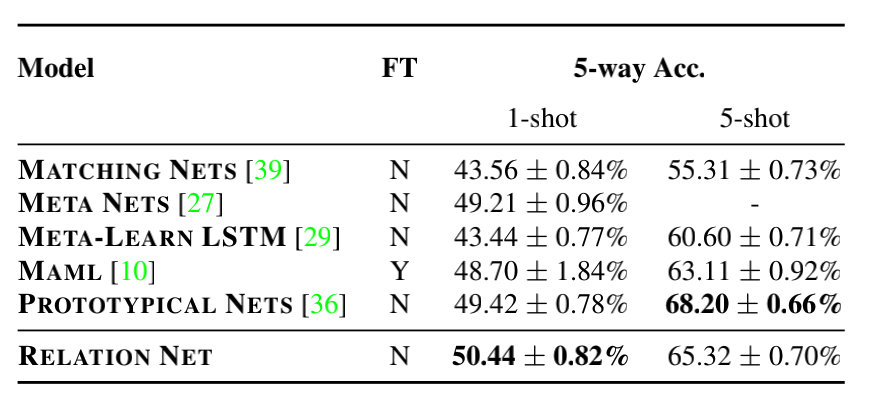5-way 1-shot 准确率：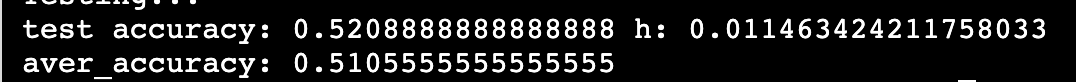5-way 5-shot 准确率：下载安装命令

## CPU版本安装命令

## GPU版本安装命令
pip install -f https://paddlepaddle.org.cn/pip/oschina/gpu paddlepaddle-gpu

GitHub:

Gitee:

END### 作者的其它热门文章

0
1 收藏

0 评论
1 收藏
0